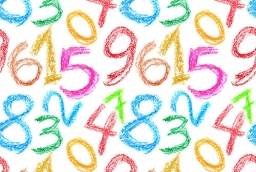# Previous 8148

The sum of three natural numbers is 204. Each of them is five greater than the previous one. Which are the numbers?

a =  63
b =  68
c =  73

### Step-by-step explanation:

a+(a+5)+(a+5+5)=204

3a = 189

a = 189/3 = 63

a = 63

Our simple equation calculator calculates it.Did you find an error or inaccuracy? Feel free to write us. Thank you!

Tips for related online calculators
Do you have a linear equation or system of equations and looking for its solution? Or do you have a quadratic equation?